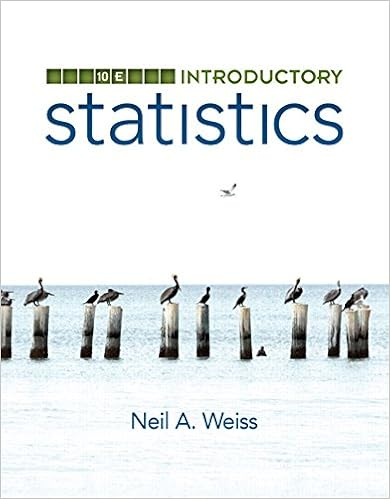# Section 10.5 - Section 10.5: Parametric Surfaces 1....

• Test Prep
• Kostilyovan
• 4

This preview shows page 1 - 2 out of 4 pages.

Section 10.5: Parametric Surfaces1.Identify the geometric object that is represented by parametric equations.a.A planee.A circleb.A conef.A circular diskc.A straight lineg.A helixd.A circular cylinderh.A sphereANS: CPTS:1
2.Identify the geometric object that is represented by parametric equations.PTS:1
3.Identify the geometric object that is represented by parametric equations.PTS:1
4.Identify the geometric object that is represented by parametric equations.PTS:1
5.Identify the geometric object that is represented by parametric equations.a.A planee.A circleb.A conef.A circular diskc.A straight lineg.A helixd.A circular cylinderh.

Course Hero member to access this document

##### We have textbook solutions for you!The document you are viewing contains questions related to this textbook.
Chapter 8 / Exercise 2
Trigonometry
McKeague/TurnerExpert VerifiedBrowse all Textbook Solutions

Course Hero member to access this document

End of preview. Want to read all 4 pages?

Course Hero member to access this document

Term
Fall
Professor
N/A
Tags
Cone, Euclidean geometry, Parametric equation, Conic section
##### We have textbook solutions for you!
The document you are viewing contains questions related to this textbook.The document you are viewing contains questions related to this textbook.
Chapter 8 / Exercise 2
Trigonometry
McKeague/TurnerExpert Verified
•••